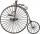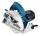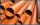# Rope

How many meters of rope 10 mm thick will fit on the bobbin diameter of 200 mm and length 350 mm (central mandrel have a diameter 50 mm)?

Result

l =  313.4 m

#### Solution:Leave us a comment of example and its solution (i.e. if it is still somewhat unclear...):

Showing 0 comments:Be the first to comment!#### To solve this example are needed these knowledge from mathematics:

Do you want to convert length units?

## Next similar examples:

1. Bicycle wheelBicycle wheel has a diameter of 70 cm. Approximately how many times the wheel rotates at 4.7 km long trip?
2. WheelDiameter of motocycle wheel is 52 cm. How many times rotates wheel on roand long 2 km?
3. SeatsSeats in the sport hall are organized so that each subsequent row has five more seats. First has 10 seats. How many seats are: a) in the eighth row b) in the eighteenth row
4. SawBlade circular saw with a diameter 42 cm turns 825 times per minute. Expresses his cutting speed in meters per minute.
5. MassThe thickness of a metallic tube is 1cm and its outer radius is 11cm. Find the mass of such 1m long tube, if the density of the metal is 7.5g per cubic cm.
6. BaseBase of building is circle with diameter 25 m. Calculate the circumference of a circular trench witch diameter is 41 cm wider than the diameter of the base.
7. TreesA certain species of tree grows an average of 0.5 cm per week. Write an equation for the sequence that represents the weekly height of this tree in centimeters if the measurements begin when the tree is 200 centimeters tall.
8. SequenceWrite the first 6 members of these sequence: a1 = 5 a2 = 7 an+2 = an+1 +2 an
9. 6 termsFind the first six terms of the sequence. a1 = 7, an = an-1 + 6
10. AS sequenceIn an arithmetic sequence is given the difference d = -3 and a71 = 455. a) Determine the value of a62 b) Determine the sum of 71 members.
11. Nineteenth memberFind the nineteenth member of the arithmetic sequence: a1=33 d=5 find a19
12. AP - simpleDetermine the first nine elements of sequence if a10 = -1 and d = 4
13. SequenceWrite the first 7 members of an arithmetic sequence: a1=-3, d=6.
14. Theorem proveWe want to prove the sentense: If the natural number n is divisible by six, then n is divisible by three. From what assumption we started?
15. Sequence 3Write the first 5 members of an arithmetic sequence: a4=-35, a11=-105.
16. Sequence 2Write the first 5 members of an arithmetic sequence a11=-14, d=-1
17. SequenceBetween numbers 1 and 53 insert n members of the arithmetic sequence that its sum is 702.